StatLect

# Parameter of a distribution

A parameter of a distribution is a number or a vector of numbers describing some characteristic of that distribution.## Examples of scalar parameters

Examples of distribution parameters are:

## Examples of vector parameters

All of the above are scalar parameters, that is, single numbers. Instead, the following are examples of vector parameters:

• the expected value of a multivariate probability distribution;

• its covariance matrix (a matrix can be thought of as a vector whose entries have been written on multiple columns/rows);

• a vector of cross-moments.

## Parametric families

You have probably read many times statements such as "Let us assume that the random variablehas a normal distribution".

What does such a statement mean?

It means thatis a continuous random variable whose probability density function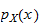can be written as: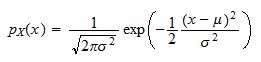wherecan be any real number and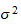can be any positive real number.

It can be proved thatandare equal to the mean and variance ofrespectively (see Normal distribution).

The two numbersandare parameters (together they form a vector parameter).

By changing them, we get different probability distributions of.

So, when we say "Let us assume that the random variablehas a normal distribution" without specifying the mean and the variance of, what we mean is "Let us assume that the distribution ofbelongs to the set of all normal distributions". This set can be obtained by varying the parametersandin the formula above, and it is called a parametric family.

## Mathematical definition of a parametric family

In mathematical terms, we have a set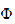of probability distributions, an we put it into correspondence with a parameter space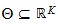.

If the correspondence is a function that associates one and only one distribution into each parameter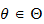, thenis called a parametric family.

In the example above,is the the set of all normal distributions, and the parameter space is the set of all couples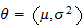such thatis positive. In other words,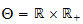. The setis a parametric family of distributions because there is only one normal distribution corresponding to a specific couple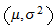.

## Examples of parametric families

Some examples of parametric families are reported in the next table.

Parametric family Distribution parameters
Bernoulli Probability of success
Binomial Probability of success and number of trials
Poisson Expected value
Uniform Upper and lower bounds of the support
Exponential Rate parameter
Normal Mean, variance
Chi square Degrees of freedom
Student's t Mean, scale parameter and degrees of freedom
Multivariate normal Expected value (vector), covariance matrix

## Model parameters

In statistical inference, we observe a sample of data and we make inferences about the probability distribution that generated the sample.

What we typically do is to set up a statistical model and carry out inferences (estimation, testing, etc.) about a model parameter.

What does it mean to set up a statistical model? It just means that we make some hypotheses about the probability distribution that generated the data, that is, we restrict our attention to a well-defined set of probability distributions (e.g., the set of all continuous distributions, the set of all multivariate normal distributions, the set of all distributions having finite mean and variance).

After setting up the model, we exploit the assumptions we have made to learn something about the distribution that generated the data.

For instance, if we have assumed that the data come from a normal distribution, we can use the observed data to estimate the distribution parameters (mean and variance) or to test the null hypothesis that one of them is equal to a specific value.

## Difference between parametric family and statistical model

The concept of a statistical model is broader than the concept of a parametric family. They are both sets of probability distributions, but the members of a model need not be uniquely identified by a parameter.

For example, suppose that our model is the set of all distributions having finite mean, and the parameter of interest, which we want to estimate, is the mean.

Then, there are several distributions in the set having the same mean: the distributions are not uniquely identified by the parameter of interest.

Actually, there is no parameter (single number or finite-dimensional vector) that allows us to uniquely identify a member of the model.

## More details

In lecture entitled Statistical inference we define parameters, parametric families and inference in a formal manner.

You can also have a look at a related glossary entry: Parameter space.

Previous entry: Null hypothesis

Next entry: Parameter space